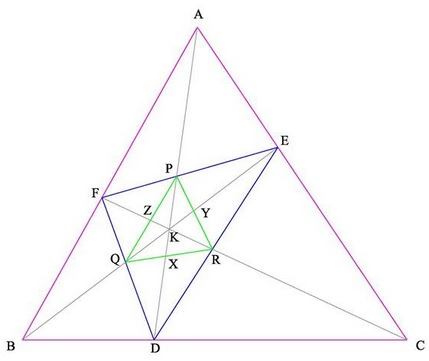# Another Identity in Triangle

Let $K$ be a point inside $\Delta ABC.$ Lines $AO,BO,CO$ cross the sides of the triangle at $D,E,F,$ and the lines $EF,DF,DE$ at $P,Q,R,$ respectively. $AD$ crosses $QR$ at $X,$ $BE$ crosses $PR$ at $Y,$ and $CF$ crosses $PQ$ at $Z.$Prove that

\begin{align}\displaystyle \frac{1}{\frac{AD}{DX}+\frac{1}{2}}+\frac{1}{\frac{BE}{EY}+\frac{1}{2}}+\frac{1}{\frac{CF}{FZ}+\frac{1}{2}}=\frac{2}{3}. \end{align}

### ProofWith $[X]$ denoting the area of figure $X,$ let $[\Delta BCK]=x,[\Delta ACK]=y, [\Delta ABK]=z.$ Previously, in a related problem, it was shown that, e.g., $\displaystyle\frac{KP}{AP}=\frac{x}{x+y+z}$ and also that $\displaystyle\frac{AK}{DK}=\frac{y+z}{x}.$ From the latter it follows that $\displaystyle\frac{DK}{AD}=\frac{x}{x+y+z},$ so that $\displaystyle\frac{KP}{AP}=\frac{DK}{AD}.$ Similarly, $\displaystyle\frac{KX}{DX}=\frac{KP}{DP}.$

On the other hand, $\displaystyle\frac{KP}{AP}=\frac{x}{x+y+z}$ implies $\displaystyle\frac{KP}{AK}=\frac{x}{2x+y+z}$ and also $\displaystyle\frac{AK}{DK}=\frac{y+z}{x}$ from which $\displaystyle\frac{AK}{AD}=\frac{y+z}{x+y+z}.$ Further,

\displaystyle\begin{align} \frac{KP}{AD}&=\frac{KP}{AK}\cdot\frac{AK}{AD}\\ &=\frac{x}{2x+y+z}\cdot\frac{y+z}{x+y+z}\\ &=\frac{x(y+z)}{(x+y+z)(2x+y+z)}. \end{align}

But $AD-AP=DP$ such that

\displaystyle\begin{align} \frac{DP}{KP}&=\frac{(x+y+z)(2x+y+z)}{x(y+z)}-\frac{x+y+z}{x}\\ &=\frac{2(x+y+z)}{y+z}. \end{align}

Thus,

$\displaystyle\frac{KX}{DX}=\frac{KP}{DP}=\frac{y+z}{2(x+y+z)}.$

From this, $\displaystyle\frac{DK}{DX}=\frac{2x+3y+3z}{2(x+y+z)}$ and, since $\displaystyle\frac{AD}{DK}=\frac{x+y+z}{x},$

\displaystyle\begin{align} \frac{AD}{DX}&=\frac{DK}{DX}\cdot\frac{AD}{DK}\\ &=\frac{2x+3y+3z}{2(x+y+z)}\cdot\frac{x+y+z}{x}\\ &=\frac{2x+3y+3z}{2x}. \end{align}

Now, $\displaystyle\frac{AD}{DX}+\frac{1}{2}=\frac{3(x+y+z)}{2x}.$ Similarly, $\displaystyle\frac{BE}{EY}+\frac{1}{2}=\frac{3(x+y+z)}{2y}$ and $\displaystyle\frac{CF}{FZ}+\frac{1}{2}=\frac{3(x+y+z)}{2z}.$ And the result follows.

### Acknowledgment

The problem has been posted by Leo Giugiuc at the CutTheKnotMath facebook page with participation of Van Khea. The proof is by Leo Giugiuc.